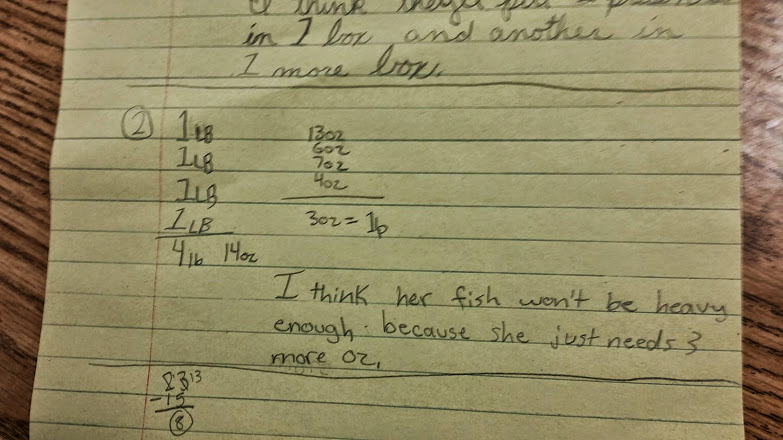# Too Heavy? Too Light? Conversions!

5 teachers like this lesson
Print Lesson

## Objective

SWBAT solve problems that require unit conversions within the same system.

#### Big Idea

In this lesson, students work independently to solve problems involving conversions and then present their strategy to their learning partner. The learning partner will then present the partner's strategy to the class.

## Number Hook

5 minutes

I start this lesson with a magic number trick I call Same Single Digit Numbers!

Students first write down two single digit numbers on a whiteboard.  I direct students to circle one of the  numbers and then double this number.  To their new sum, I then direct students to add 5.  Next, multiply by 5. Next, students add the second single digit number to the new sum.  Last, students subtract 4. Next subtract 21 and you end up with the starting digits.

Example with 6 and 8

6 x 2= 12

12 + 5 = 17

17 *5  = 85

85 + 8 = 93

93 - 4 = 89

89 - 21 =  68   (the first two digits I started with)

## Concept Development

50 minutes

In this lesson, students work independently to solve three problems involving metric and customary mass.  To begin this lesson, I give each student a copy of the three problems. too light too heavy.  Next, I explain to students that they will have about 30 minutes to complete the three problems.

For the problems, students are asked to combine several weights to find the total weight. Since all weights are given using two different units (kg and g, or lb and oz), students must think about  how the units are related to each other. For example, students will need to use the following relationships: 1 kg = 1,000 g and 1 lb = 16 oz. Students may use different strategies to answer the questions on the “Too Heavy? Too Light?” student recording sheet. Some may choose to convert everything to the smaller unit (g or oz) before adding the given weights. Others will try to add the two units separately and then convert, if necessary.

You can see one student's work example for problem 2 below.  You can see this student's strategy is to add up all the pounds in one column and add the ounces in another column.You can hear in this video below another student using a very similar strategy for the first problem

When most students are finished, I direct students to look back at the first problem.  Their job is to talk for three minutes with their learning partner about HOW they solved the problem and HOW they reached their answer.

This video shows a quick snap shot of my students working independently before they begin talking with their partners.

In this video, you can hear and see a student explaining his thinking and answer to his partner.

In this video, you can hear and see the other partner from the above video talking about her strategy and making comparisons with her partner.

After partners have had an opportunity to share with each other, I lead a brief discussion with the entire class.  I go over the correct answers, but spend time with each problem discussing strategies with the class, emphasizing the HOW and WHY of each problem, rather than the correct answers.